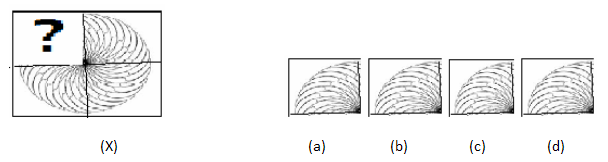# Pattern Completion - Non Verbal Reasoning questions

## Pattern Completion - Non Verbal Reasoning questions

1. Complete the pattern in fig. (X) by selecting one of the figures from the four alternatives.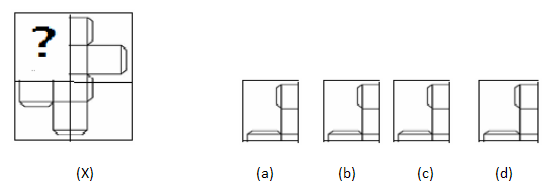2. Complete the pattern in fig. (X) by selecting one of the figures from the four alternatives.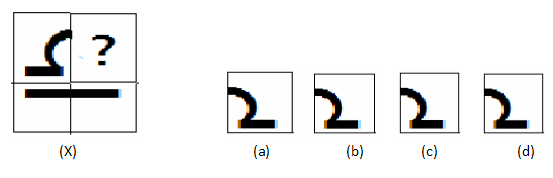3. Complete the pattern in fig. (X) by selecting one of the figures from the four alternatives.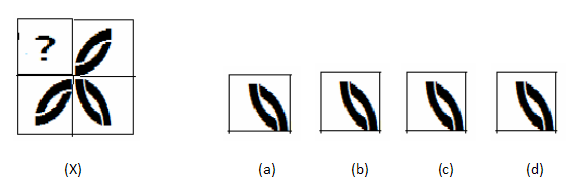4. Complete the pattern in fig. (X) by selecting one of the figures from the four alternatives.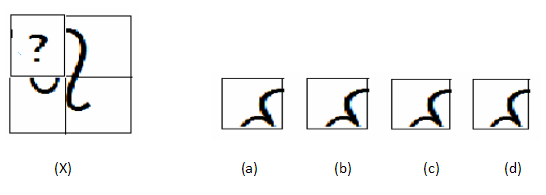5. Complete the pattern in fig. (X) by selecting one of the figures from the four alternatives.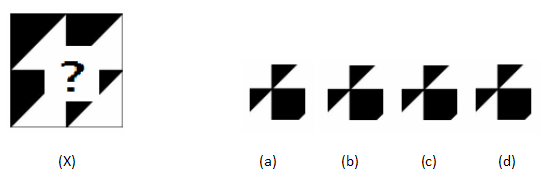6. Complete the pattern in fig. (X) by selecting one of the figures from the four alternatives.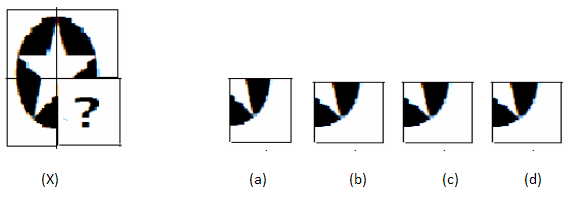7. Complete the pattern in fig. (X) by selecting one of the figures from the four alternatives.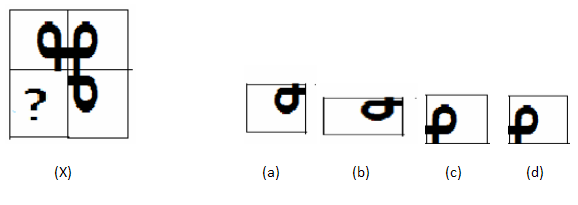8. Complete the pattern in fig. (X) by selecting one of the figures from the four alternatives.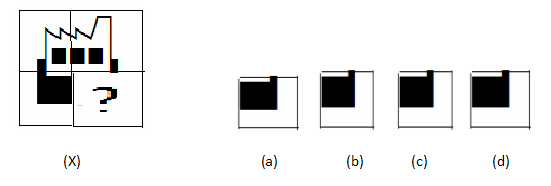9. Complete the pattern in fig. (X) by selecting one of the figures from the four alternatives.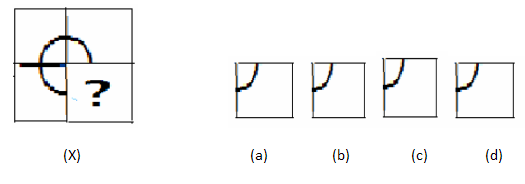10. Complete the pattern in fig. (X) by selecting one of the figures from the four alternatives.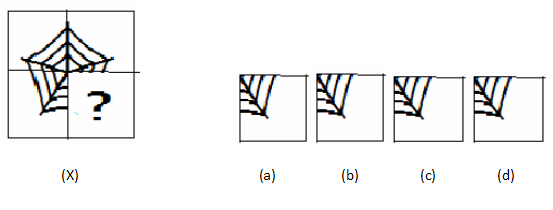11. Complete the pattern in fig. (X) by selecting one of the figures from the four alternatives.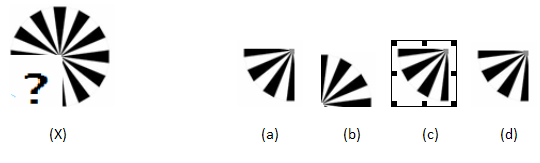12. Complete the pattern in fig. (X) by selecting one of the figures from the four alternatives.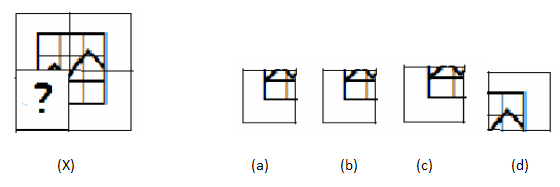13. Complete the pattern in fig. (X) by selecting one of the figures from the four alternatives.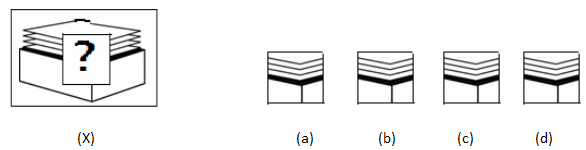14. Complete the pattern in fig. (X) by selecting one of the figures from the four alternatives.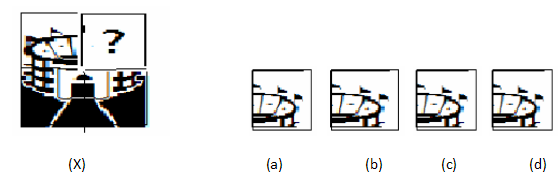15. Complete the pattern in fig. (X) by selecting one of the figures from the four alternatives.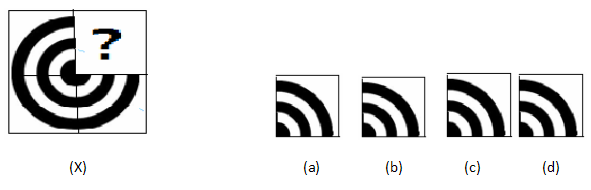16. Complete the pattern in fig. (X) by selecting one of the figures from the four alternatives.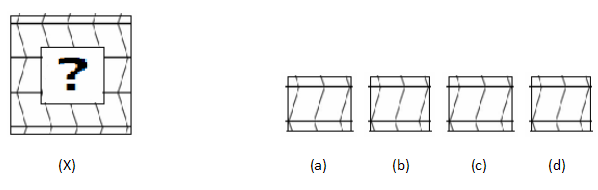17. Complete the pattern in fig. (X) by selecting one of the figures from the four alternatives.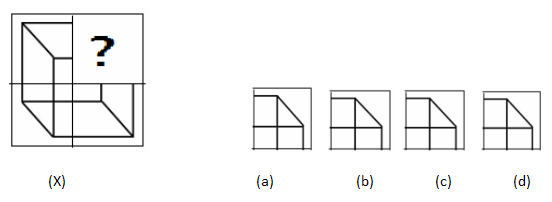18. Complete the pattern in fig. (X) by selecting one of the figures from the four alternatives.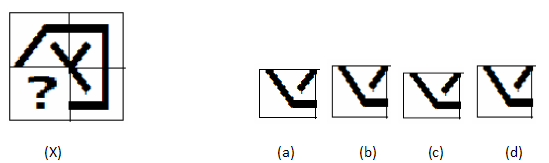19. Complete the pattern in fig. (X) by selecting one of the figures from the four alternatives.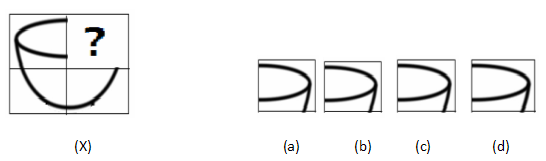20. Complete the pattern in fig. (X) by selecting one of the figures from the four alternatives.